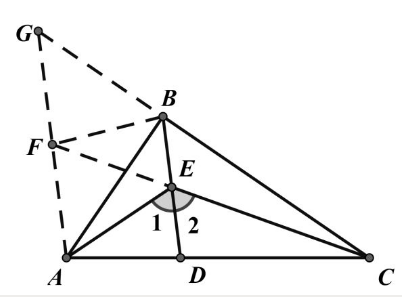【答案】

$$F A=F G=F B \text {, }$$

\begin{aligned} & \angle A D E=\angle F B E \\ & \angle C B E=\angle B G F=\angle G B F \\ & \angle F B A=\angle A B D \\ & \therefore \angle A D B=2 \angle A B D 。 \end{aligned}